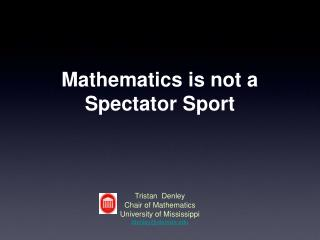Download PresentationMathematics is not a Spectator Sport

# Mathematics is not a Spectator Sport

Download Presentation## Mathematics is not a Spectator Sport

- - - - - - - - - - - - - - - - - - - - - - - - - - - E N D - - - - - - - - - - - - - - - - - - - - - - - - - - -
##### Presentation Transcript

1. Mathematics is not a Spectator Sport • Tristan Denley • Chair of Mathematics • University of Mississippi • tdenley@olemiss.edu

2. PreliminaryStudy • Hawkes Learning System • 2 Fall sections of College Algebra • 100 students • MyMathlab • 2 Fall sections of College Algebra • 113 students • No software • 4 fall sections of College Algebra • 200 students

3. Final Exams College Algebra

4. Final Exams College Algebra

5. C.A.L.M. Computer lab • 80 Computers • Open 60 hours each week • Staffed with instructors and graduate students • Students have required lab and lecture time

6. Final Exams Elementary Statistics

7. Final Exams Elementary Statistics ~1300 students in each cohort

8. What have we gained? • Fall 2000 • College Algebra 966 students • Elementary Statistics 307 students • 26 Instructors • Fall 2007 • College Algebra 1070 students • Elementary Statistics 864 students • Not 39 Instructors - 25 instructors

9. The hidden story • Fall 2000 • Discrete Mathematics • Introduction to Linear Algebra • Elementary Differential Equations • Modern Algebra I • History of Mathematics • Advanced Calculus I • Spring 2001 • Applied Modern Algebra • Foundations of Mathematics • Elementary Differential Equations • Intermediate Differential Equations • Modern Algebra II • Advanced Calculus II • Applied Probability I • Fall 2007 • Discrete Mathematics • Foundations of Mathematics • Introduction to Linear Algebra • Elementary Differential Equations • Introduction to Statistical Methods • Techniques in Teaching Sec. Level Math • Introduction to Complex Analysis • Introduction to Acturial Science • Modern Algebra I • Topics in Euclidean Geometry • History of Mathematics • Advanced Calculus I • Mathematical Statistics I • Spring 2008 • Discrete Mathematics • Applied Modern Algebra • Foundations of Mathematics • Introduction to Linear Algebra • Elementary Differential Equations • Introduction to Statistical Methods • Combinatorics • Introduction to Abstract Algebra • Introduction to Mathematical Statistics • Topology • Number Theory • Modern Algebra II • Advanced Calculus II • Mathematical Statistics II • Applied Multiple Regression

10. The hidden story • 2006-2007 • 81 Math Majors • 50 BA students • 31 BS students • 20 PhDs over the last 4 years • 2000-2001 • 45 Math Majors • 40 BA students • 5 BS Students • PhD program put on probation

11. Thank you Tristan Denley Chair of Mathematics University of Mississippi tdenley@olemiss.edu

12. Course Redesign • Course redesign is the process of redesigning whole courses (rather than individual classes or sections) to achieve better learning outcomes. • Course redesign is NOT just about putting courses online. It is about rethinking the way we deliver instruction

13. Why Redesign? • Accommodate more students without adding resources and without sacrificing standards. • Free up faculty members to offer other courses and programs of study that are in demand. • Improve consistency and quality across multiple sections.

14. Final Exams Intermediate Algebra

15. Grades by Gender College Algebra

16. Those who took the final Those who took the final Mean Pass % Spring 2007 68.2 Spring 2007 61.1 Spring 2006 72.6 Spring 2006 64.1 Final Exams Intermediate Algebra